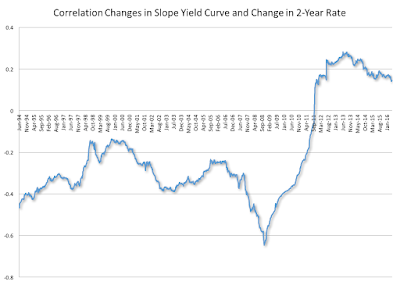Tuesday, May 31, 2016

How informative is the slope of the Yield Curve?

The yield curve is becoming flatter. The difference between the 10-year and 2-year government bond is now approaching 1%. The yield curve tends to get flatter when the economy reaches the end of an expansion phase and it is many times seen as a predictor of future recessions.But interest rates are not what they used to be. If short-term interest rates are stuck at zero, all the movements in the yield have to come from long-term interest rates. This is the opposite than what we have seen in previous cycles where all the action has come from short term rates.

The 2-year rate is not quite zero and has been moving recently, so an interesting question is whether the yield curve is once again driven by movements in short-term rates. Not quite. Let's calculate the correlation between changes in the slope  of the yield curve (measured as 10 year minus 2 year rates) and the changes in the 2 year rate. The correlation [calculated over a 3 year window] is plotted below.If the 10 year rate did not move and all the action was coming from the 2 year rate this correlation would be -1. Prior to 2011 this correlation was always negative and in some cases as high as -0.6 signaling the importance of changes in short-term rates as drivers of changes in the slope of the yield curve.

But as of the summer of 2011 this correlation has turned positive indicating the importance of movements in the long-term rates and the way they are correlated with the 2-year rate. As an illustration: an event that causes both short-term and long-term rates to move down but where the 10 year rate falls by more would lead to a positive correlation between changes in short-term rates and changes in the slope of the yield curve.

So we are living in a new world where change in the slope of the yield curve are driven by a combination of changes in both short-term and long-term rates that are not easily mapped into previous cycles. Where the yield curve goes from here is an open question. A strong recovery and an increase in inflation expectations could result in an increase in its slope and possibly the return towards more normal times. A negative event could potentially lead to a further flattening of the yield curve and one more step towards Japanification of the US economy.

Antonio Fatás